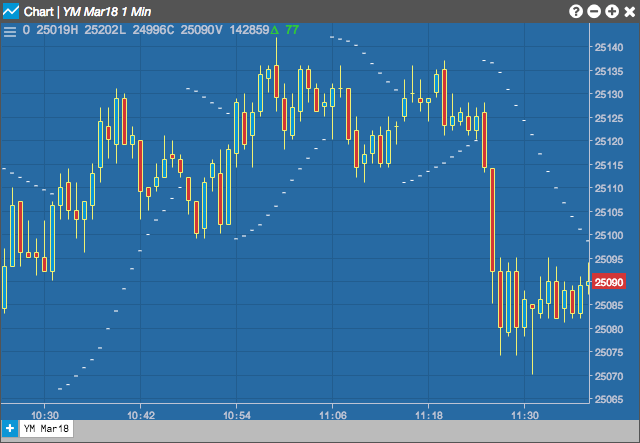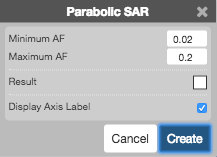Charts

# Parabolic Sar (SAR)

The Parabolic Stop and Reverse (SAR) calculates trailing stop points to use with long and short positions. The SAR was published by J. Welles Wilder as part of a complete trend following system. The dotted lines above the price designate trailing stops for short positions; those below the price are sell stops for long positions.## Configuration Options• Minimum AF: Minumum acceleration factor
• Maximum AF: Maximum acceleration factor
• Color Selectors: Colors to use for graph elements.
• Display Axis Label: Whether to display the most recent value on the Y axis.

## Formula

For new long positions:

$SAR = P + (A * ( H - P ))$

Where:

• SAR = the long Stop and Reverse price at which the position is reversed from Long to Short
• P = the previous period's SAR
• A = the acceleration factor.
• H = the highest price since the current long trade was opened on a buy stop order.

For new short positions:

$SAR = P - (A * ( L - P ))$

Where:

• S = the short side buy Stop and Reverse Price (SAR) at which the position is reversed from short to long
• P = the previous period's SAR
• A = the acceleration factor.
• L = the lowest price since the current short trade was opened on a sell stop order.